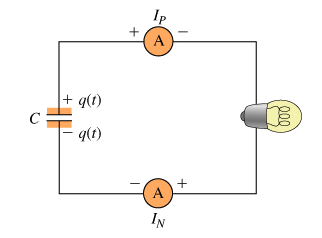# Problem: A large capacitor has a charge +q on one plate and -q on the other. At time t=0, the capacitor is connected in series to two ammeters and a light bulb. Immediately after the circuit is closed, the ammeter connected to the positive plate of the capacitor reads IP and the ammeter connected to the negative plate of the capacitor reads IN. (Figure 1)Each ammeter reads positive if current flows through the circuit in a clockwise direction (from the + to the – terminal of the meter).Immediately after time t = 0, what happens to the charge on the capacitor plates?Check all that apply.a. Individual charges flow through the circuit from the positive to the negative plate of the capacitor.b. Individual charges flow through the circuit from the negative to the positive plate of the capacitor.c. The positive and negative charges attract each other, so they stay in the capacitor.d. Current flows clockwise through the circuit.e. Current flows counterclockwise through the circuit.

###### FREE Expert Solution

In circuits:

• Negative charges move from the negative plate to the positive plate.
• Conventional current moves from the positive plate to the negative plate.

Combining the two points above, charges and electric current move in opposite directions.

88% (391 ratings)###### Problem Details

A large capacitor has a charge +q on one plate and -q on the other. At time t=0, the capacitor is connected in series to two ammeters and a light bulb. Immediately after the circuit is closed, the ammeter connected to the positive plate of the capacitor reads IP and the ammeter connected to the negative plate of the capacitor reads IN. (Figure 1)

Each ammeter reads positive if current flows through the circuit in a clockwise direction (from the + to the – terminal of the meter).Immediately after time t = 0, what happens to the charge on the capacitor plates?

Check all that apply.

a. Individual charges flow through the circuit from the positive to the negative plate of the capacitor.

b. Individual charges flow through the circuit from the negative to the positive plate of the capacitor.

c. The positive and negative charges attract each other, so they stay in the capacitor.

d. Current flows clockwise through the circuit.

e. Current flows counterclockwise through the circuit.